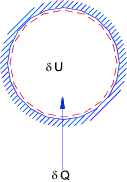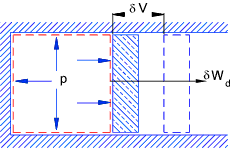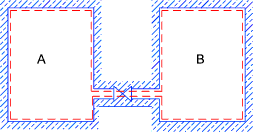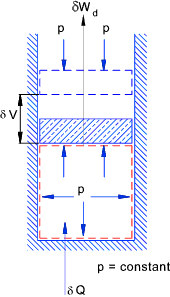Thermos Index

First Law of Thermodynamics
Non-Flow Processes

 Introduction The intrinsic internal energy U is the total internal energy minus the energies of motion, gravitational, magnetic and surface forces energies .  The first law can be written using U as δQ = δU + δW This is termed the restricted energy conservation equation for a system   The notes below relate to various non flow processes using the restricted energy conservation equation when the kinetic and potential and surface energies are assumed zero.   The system boundaries are defined by the red dashed lines. Heating at constant volumeδW= 0 therefore δQ= δU Adiabetic expansion in a cylinderδQ= 0 therefore δW= -δU Free Expansion (Joules experiment)Initially valve is closed and pA > pB > Valve is opened and pressures equalise.( Q = W = 0) E is constant throughout process When conditions settle U = E therefore final U = initial U Throughout path of proces U is not constant. Heating at constant pressureδQ= δU + δW If the expansion is fully resisted (reversible process) δW= pδV therefore δQ= δH Note: δH = δU + pδV + Vδp.  In the above system p is constant (δp = 0). Therefore δH = δU + pδV

 Thermodynamic Law Links Thermodynamics..NASA - Glenn Research center at Series of informative notes on Thermodynamics Second Law of Thermodynamics..Interesting Article

Thermodynamic Laws